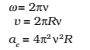In this section, we shall see how to describe motion in two dimensions using vectors.

## Position Vector and Displacement:

The position vector r of a particle P located in a plane with reference to the origin of an x-y reference frame (Fig.4. 12 ) is given by
r = xî + yĵ
where x and y are components of r along x-, and y- axes or simply they are the coordinates of the object.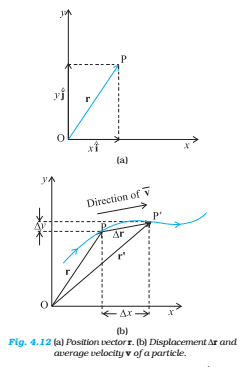Suppose a particle moves along the curve shown by the thick line and is at P at time t and P′ at time t′ [Fig. 4.12(b)]. Then, the displacement is :

∆r = r’ – r

and is directed from P to P′ .

Component form: ∆r = (xî + yĵ ) – (xî + yĵ )

= ∆xî+ ĵ∆y

Where ∆x = x’ – x , ∆y = y’ – y

### Velocity:

The average velocity () of an object is the ratio of the displacement and the corresponding time interval: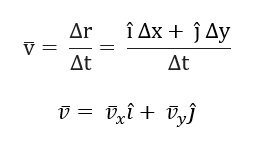Since,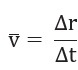the direction of the average velocity is the same as that of Δr.

The velocity (instantaneous velocity) is given by the limiting value of the average velocity as the time interval approaches zero: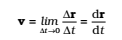Therefore, the direction of velocity at any point on the path of an object is tangential to the path at that point and is in the direction of motion.

### Acceleration:

The average acceleration a of an object for a time interval Δt moving in x-y plane is the change in velocity divided by the time interval: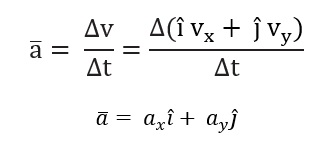The acceleration (instantaneous acceleration) is the limiting value of the average acceleration as the time interval approaches zero: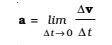Note: that in one dimension, the velocity and the acceleration of an object are always along the same straight line (either in the same direction or in the opposite direction). However, for motion in two or three dimensions, velocity and acceleration vectors may have any angle between 0° and 180° between them.

## Relative velocity in two Dimensions

The concept of relative velocity, introduced in for motion along a straight line, can be easily extended to include motion in a plane or in three dimensions. Suppose that two objects A and B are moving with velocities vA and vB
(each with respect to some common frame of reference, say ground.). Then, velocity of object A relative to that of B is:

VAB = VA – VB

Similarly, the velocity of object B relative to that of A is:

VBA = VB – VA

Therefore, VAB = -VBA

And , |VAB|= |VBA|

## Projectile Motion

An object that is in flight after being projected is called a projectile. If an object is projected with initial velocity v0 making an angle θ0 with x-axis and if we assume its initial position to coincide with the origin of the coordinate system, then the position and velocity of the projectile at time t are given by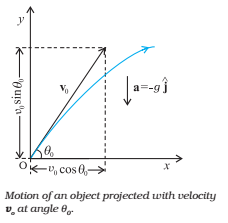x = (v0 cosθ0) t

y = (v0 sinθ0) t – (1/2) g t2

vx = v0x = v0 cosθ0

vx = v0 sinθ0 –g t

The path of a projectile is parabolic and is given by: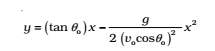The maximum height that a projectile attains is: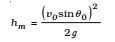The time taken to reach this height is:

The horizontal distance travelled by a projectile from its initial position to the position it passes y = 0 during its fall is called the range, R of the projectile. It is: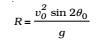If R is maximum when sin 2θ0 is maximum, i.e., when θ0 = 450. The maximum horizontal range is, therefore,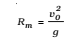## Uniform Circular Motion

When an object follows a circular path at constant speed, the motion of the object is called uniform circular motion.

The magnitude of its acceleration is: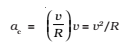The acceleration of an object moving with speed v in a circle of radius R has a magnitude and is always directed towards the centre. This is why this acceleration is called centripetal acceleration (a term proposed by Newton).

The direction of ac is always towards the centre of the circle. The angular speed ω, is the rate of change of angular distance.

It is related to velocity v by v = ωR.

The acceleration is ac = ω2R.

If T is the time period of revolution of the object in circular motion and ν is its frequency, we have